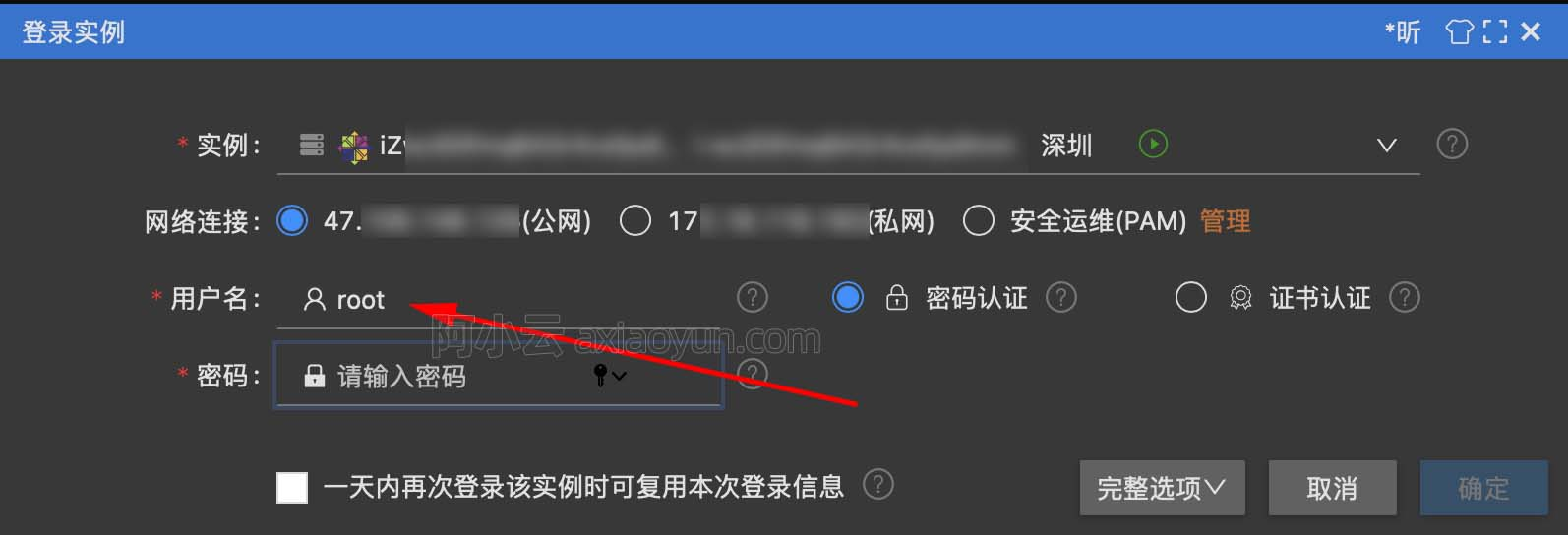# word2vec深入浅出，保证你一看就会（2）

+关注继续查看

milkov2013的文章很难以理解，有以下论文对这篇论文进行了详细解读精读！
http://www-personal.umich.edu/~ronxin/pdf/w2vexp.pdf

## skip-gram的计算问题input->projection层实际上就是取了一列（或者一行），实际计算量为 H, H为hidden层个数，也就是word的vec维度
projection->output层计算为$$hw2$$,上期中提到过$$h$$是H维的向量，$$w2$$是HV维的矩阵，因此这个计算要花费H*V次。

projection->output层

$$w2^{new}_{ij}=w2^{old}_{ij}-η*(输出层的错误_i)*h_j$$

## 解决方法

#### 代码分析

         if (negative > 0) for (d = 0; d < negative + 1; d++) {
if (d == 0) {
target = word;
label = 1;
} else {
next_random = next_random * (unsigned long long)25214903917 + 11;
target = table[(next_random >> 16) % table_size];
if (target == word) continue;
label = 0;
}

for循环中，d=0的时候，是指代正样本的更新，word存储了output的one-hot表示中1所在列号（维）。
target就存储了这个列（维）号，label则存储了output的one-hot在这一列（维）的值。正样本时候就是1.
d不是0的时候，随机选择负样本列（维），next_random随机了一个数，然后mod table_size(就是mod V词汇量)，如果target的数值是word（即正样本列号）就重新随机负样本列，label是0。

          l2 = target * layer1_size;
f = 0;
for (c = 0; c < layer1_size; c++) f += neu1[c] * syn1neg[c + l2];
g=输出层error;
for (c = 0; c < layer1_size; c++) neu1e[c] += g * syn1neg[c + l2];
for (c = 0; c < layer1_size; c++) syn1neg[c + l2] += g * neu1[c];

forward :

$$o=h*w2_{.target}$$

back,derivation for h:

$$h^{new}=h^{old}-η*输出层的错误*w2_{.target}$$

back,derivation for w2 column "target":

$$w2^{new}_{.target}=w2^{old}_{.target}-η*输出层的错误*h$$

l2 = target * layer1_size;

for (c = 0; c < layer1_size; c++) f += neu1[c] * syn1neg[c + l2];

for (c = 0; c < layer1_size; c++) neu1e[c] += g * syn1neg[c + l2];

for (c = 0; c < layer1_size; c++) syn1neg[c + l2] += g * neu1[c];

## NEGATIVE SAMPLING为什么能得到好的效果

NEGATIVE SAMPLING看上去非常水，竟然随机取了几列更新，这能行？2375 04558 0Xshell使用SSH远程登录阿里云ECS服务器CentOS7
10241 08043 09476 0word2vec深入浅出，保证你一看就会（2）

3738 0word2vec深入浅出，保证你一看就会（5）【完结】

3015 0word2vec深入浅出，保证你一看就会（3）

2139 01152 03457 0
6

0

《SaaS模式云原生数据仓库应用场景实践》

《看见新力量：二》电子书